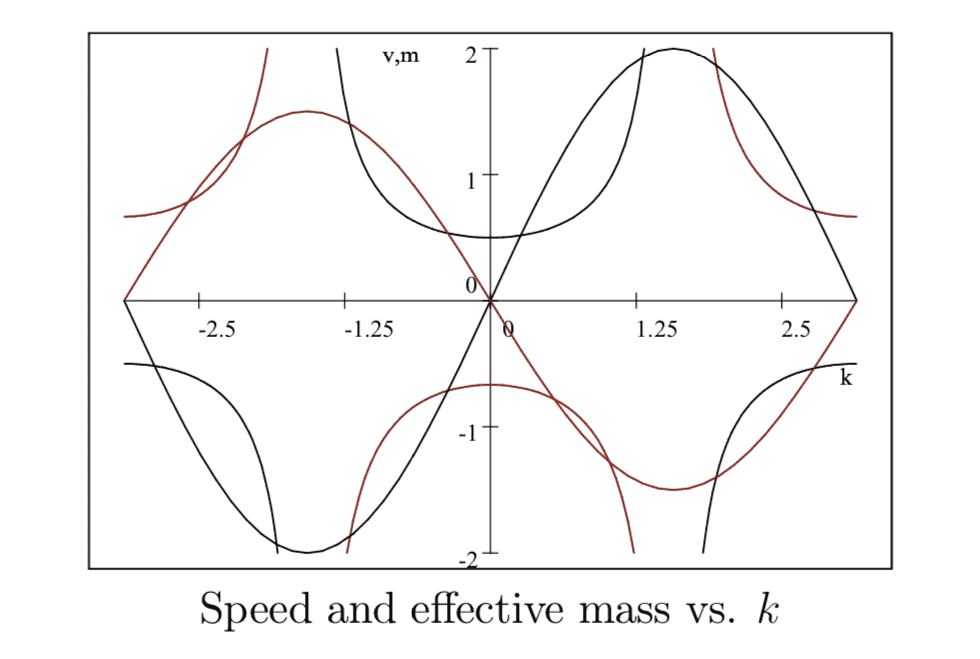# Periodic electron motion in a perfect conductor using a semiclassical model

• I
Gold Member
Summary:
Imagine to have a perfect conductor, i.e. no impurities and no thermal motion (0K)
According to the semiclassical approximation, in response to a constant electric field I would get a periodic motion of the electron, like this:The sinusoidal type function is the velocity, while the function that goes to infinity is the effective mass. Thus I was wondering, since ##v## also gets negative values, does it means that an electron oscillates back and forth ?

Thanks,
Ric

Last edited:

fluidistic
Gold Member
•dRic2
Gold Member
Yes, this is called Bloch oscillations.

Thanks! Does the electron lose energy due to radiation emission ?

•jim mcnamara and fluidistic
fluidistic
Gold Member
Thanks! Does the electron lose energy due to radiation emission ?
Good question. I think not, because radiation is a surface phenomenon while we are considering a perfect crystal (and at 0 K), i.e. a bulk. But then it's very strange, because we have a time varying current (AC), but no radiation. I'm not sure what's going on.

•dRic2
Gold Member
Good question. I think not, because radiation is a surface phenomenon while we are considering a perfect crystal (and at 0 K), i.e. a bulk. But then it's very strange, because we have a time varying current (AC), but no radiation. I'm not sure what's going on.
That's reasonable. At least it is the only think I can think of... I am dumber than usual when it comes to radiation, but assuming that there is a continuous exchange of radiation between electrons in the bulk, wouldn't you expect a more complicated motion instead of simple oscillations ?

fluidistic
Gold Member
That's reasonable. At least it is the only think I can think of... I am dumber than usual when it comes to radiation, but assuming that there is a continuous exchange of radiation between electrons in the bulk, wouldn't you expect a more complicated motion instead of simple oscillations ?
Yeah for sure. For one, Bloch oscillations are derived from the idependant electrons assumption.

•dRic2
Gold Member
Yeah for sure. For one, Bloch oscillations are derived from the idependant electrons assumption.
Then I find very surprising that they have been detected experimentally